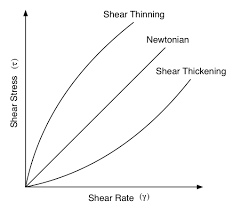# How to Calculate and Solve for Viscosity of Pseudoplastic Fluids | RheologyThe image above represents viscosity of pseudoplastic fluids.

To compute for viscosity of pseudoplastic fluids, three essential parameters are needed and these parameters are Flow Index (n), Shear Rate (γ) and Consistency (ηp).

The formula for calculating viscosity of pseudoplastic fluids:

ηo = ηp γn – 1

Where:

ηo = Viscosity of Pseudoplastics Fluids
n = Flow Index
γ = Shear Rate
ηp = Consistency

Let’s solve an example;
Find the viscosity of pseudoplastic fluids when the flow index is 20, the shear rate is 14 and the consistency is 10.

This implies that;

n = Flow Index = 20
γ = Shear Rate = 14
ηp = Consistency = 10

ηo = ηp γn – 1
ηo = 10 x 1420 – 1
ηo = 10 x 1419
ηo = 10 x 5.97e+21
ηo = 5.97

Therefore, the viscosity of pseudoplastic fluids is 5.97e+22.

Nickzom Calculator – The Calculator Encyclopedia is capable of calculating the viscosity of pseudoplastic fluids.

To get the answer and workings of the viscosity of pseudoplastic fluids using the Nickzom Calculator – The Calculator Encyclopedia. First, you need to obtain the app.

You can get this app via any of these means:

To get access to the professional version via web, you need to register and subscribe for NGN 2,000 per annum to have utter access to all functionalities.
You can also try the demo version via https://www.nickzom.org/calculator

Apple (Paid) – https://itunes.apple.com/us/app/nickzom-calculator/id1331162702?mt=8
Once, you have obtained the calculator encyclopedia app, proceed to the Calculator Map, then click on Materials and Metallurgical under Engineering.Now, Click on Rheology under Materials and MetallurgicalNow, Click on Viscosity of Pseudoplastic Fluids under RheologyThe screenshot below displays the page or activity to enter your values, to get the answer for the viscosity of pseudoplastic fluids according to the respective parameters which is the Flow Index (n), Shear Rate (γ) and Consistency (ηp).Now, enter the values appropriately and accordingly for the parameters as required by the Flow Index (n) is 20, Shear Rate (γ) is 14 and Consistency (ηp) is 10.Finally, Click on CalculateAs you can see from the screenshot above, Nickzom Calculator– The Calculator Encyclopedia solves for the viscosity of pseudoplastic fluids and presents the formula, workings and steps too.